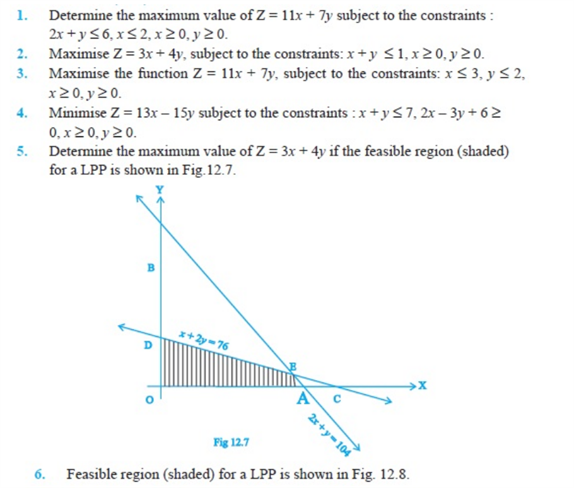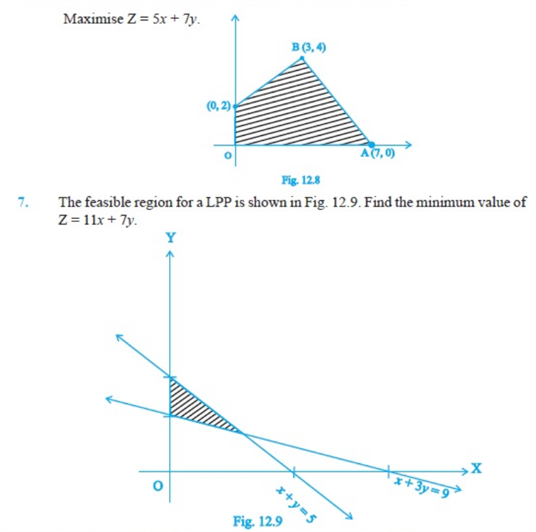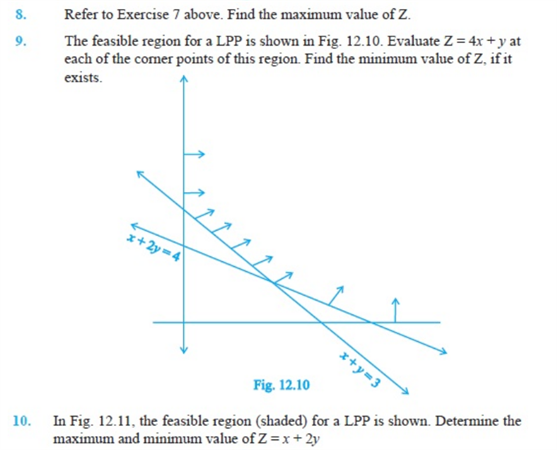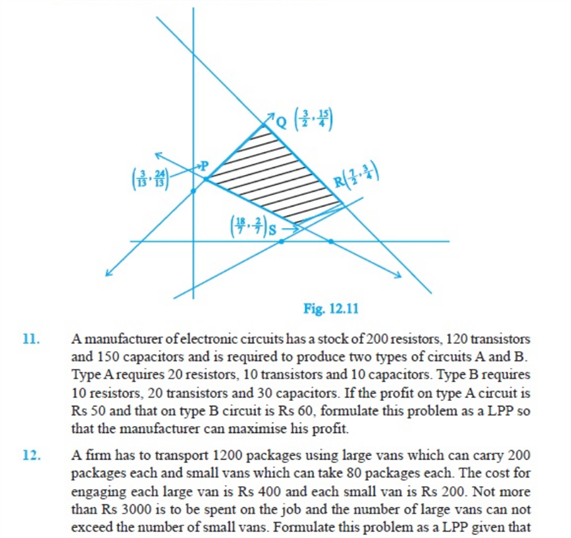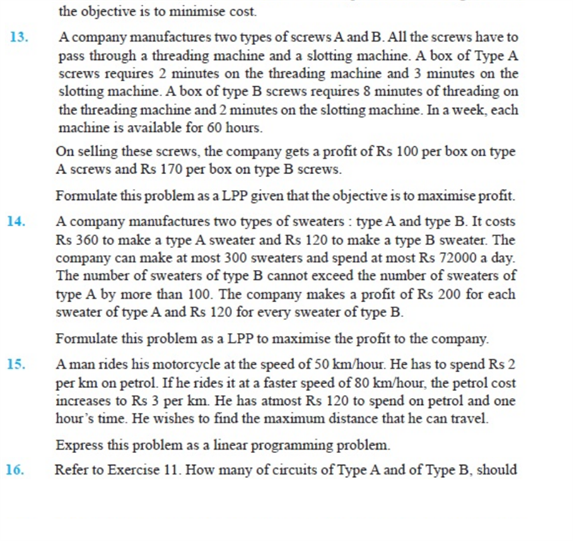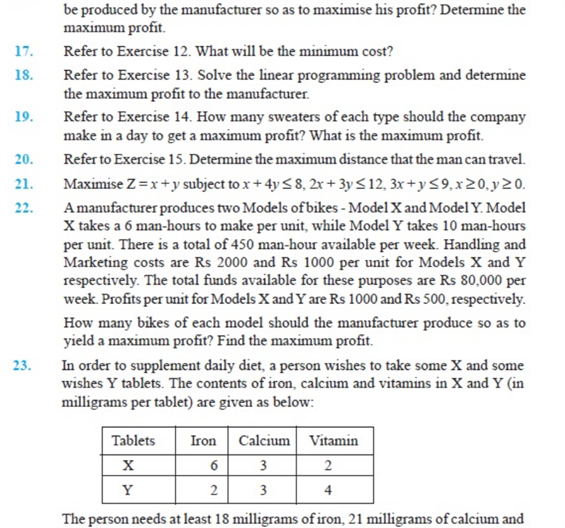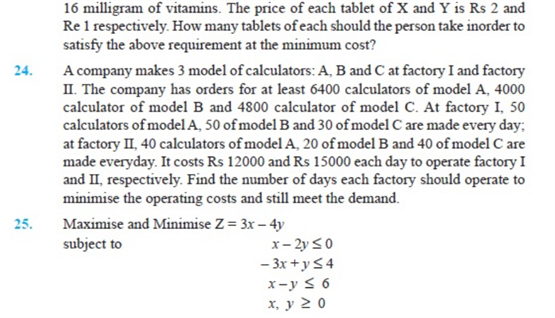# Chapter 12 - Linear Programming

Chapter 12 Linear Programming class 12 is an important chapter. It involves the linear inequations which have two variables. Solving these chapter 12 Linear Programming questions will help you prepare well for Class 12 CBSE Board exams. Also, find the NCERT Questions onto understand the topic better.

Also, Check

## Important Questions on Linear Programming Class 12 (Chapter 12)

Question 1) Graph the solution set of the following inequality:

a ≥ b – 2

Question 2) Graph the solution set of the inequation 2a – b  ≥ 1.

Question 3) If a young man rides his motorcycle at 25 km per hour, he has to spend Rs 2 per kilometre on petrol on petrol; if he rides it at a faster speed of 40 km per hour, the petrol cost increases to Rs 5 per kilometre. He has rs 100 to spend on petrol and wishes to find the maximum distance he can travel within one hour. Express this as a linear programming problem and then solve it.

Question 4)  Maximize Z = 3x + 5y, subject to the constraints;

x + 2y ≤  2000, x + y ≤  1500, y ≤  600, x

Question 5) Maximize z = 60 a +15 b, subject to the constraints

a + b ≤ 50, 3a + b ≤ 90, a,b  ≥0.

### NCERT Questions on Linear Programming Chapter 12 (Class 12)